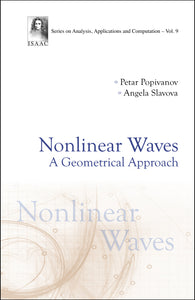# Nonlinear Waves: A Geometrical Approach

Format: Print Book

ISBN: 9789813271609

• SGD 139.10
Unit price per
Tax included.

This volume provides an in-depth treatment of several equations and systems of mathematical physics, describing the propagation and interaction of nonlinear waves as different modifications of these: the KdV equation, Fornberg-Whitham equation, Vakhnenko equation, Camassa-Holm equation, several versions of the NLS equation, Kaup-Kupershmidt equation, Boussinesq paradigm, and Manakov system, amongst others, as well as symmetrizable quasilinear hyperbolic systems arising in fluid dynamics.Readers not familiar with the complicated methods used in the theory of the equations of mathematical physics (functional analysis, harmonic analysis, spectral theory, topological methods, a priori estimates, conservation laws) can easily be acquainted here with different solutions of some nonlinear PDEs written in a sharp form (waves), with their geometrical visualization and their interpretation. In many cases, explicit solutions (waves) having specific physical interpretation (solitons, kinks, peakons, ovals, loops, rogue waves) are found and their interactions are studied and geometrically visualized. To do this, classical methods coming from the theory of ordinary differential equations, the dressing method, Hirota's direct method and the method of the simplest equation are introduced and applied. At the end, the paradifferential approach is used.This volume is self-contained and equipped with simple proofs. It contains many exercises and examples arising from the applications in mechanics, physics, optics and, quantum mechanics.

Format: Hardcover
No of Pages: 208
Imprint: World Scientific
Publication date: 20181119
Series: Series On Analysis, Applications And Computation